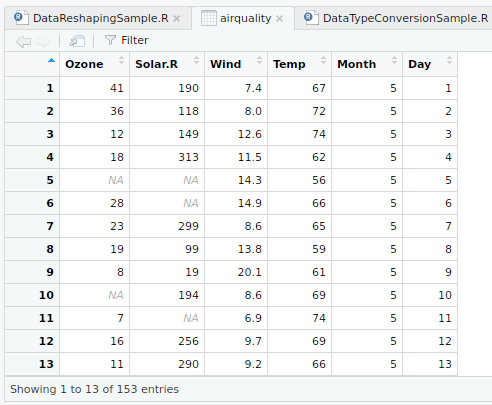• #5, First Floor, 4th Street Dr. Subbarayan Nagar Kodambakkam, Chennai-600 024 Landmark : Samiyar Madam
• pro@slogix.in
• +91- 81240 01111

### How to find optimal number of clusters using NbClust package in R?

###### Description

To find the optimal number of clusters using NbClust package in R.

###### Process
• R Package : factoextra– For data
manipulation and visualization.
• R Package : NbClust– For Visualizing
the number of clusters
• R Function : fviz_nbclust(x,FUNcluster,
method=c(“silhoutte”,”wss”,”gap_stat”))
from factoextra package used to compute

three different methods(silhoutte,
elbow,gap statistic) for any partitioning
clustering methods(k-means,k-mediods,
HCUT)

• R Function : NbClust(data, min.nc= ,
max.nc= ,method= ,distance= ) –
– from NbClust package can simultaneously

computes all the indices and determine the
number of clusters in a single function call.

• nc-minimum number of clusters
• nc -maximum number if clusters
• method- kmeans- for kmeans clustering

“ward.D”, “ward.D2”, “single”, “complete”,
“average” – for hierarchical clustering

• distance — “euclidean”, “manhattan”
or “NULL”.
###### Sapmle Code

#Optimal Number of Clusters

#install.packages(“factoextra”)
library(“factoextra”)
#install.packages(“NbClust”)
library(“NbClust”)

#Input dataset
input View(input)

#Elbow method
fviz_nbclust(input[,3:4],kmeans,method = “wss”) +
geom_vline(xintercept = 3,linetype=2) +
labs(subtitle=”Elbow Method”)

#Silhouette Method
fviz_nbclust(input[,3:4],kmeans,method = “silhouette”) +
labs(subtitle=”Silhouette Method”)

#Gap Statistic method
fviz_nbclust(input[,3:4],kmeans,method = “gap_stat”)
labs(subtitle=”Gap Statistic Method”)

#Nbclust() function to find optimal no of clusters using 30 methods at a time
NbClust(input[,3:4], min.nc = 2, max.nc = 10,method = “kmeans”, distance = “euclidean”)

###### Screenshots